# Optimal Vent Design for Additional Sunspace of Passive Houses in Rural Areas

Optimal Vent Design for Additional Sunspace of Passive Houses in Rural Areas
Hebei University of Science and Technology, Shijiazhuang 050000, China

School of Civil Engineering, Hebei University of Science and Technology, Shijiazhuang 050000, China

Corresponding Author Email:
0211708005@stu.hebust.edu.cn
Page:
1163-1170
|
DOI:
https://doi.org/10.18280/ijht.370426
5 April 2019
|
Accepted:
20 September 2019
|
Published:
26 December 2019
| Citation

OPEN ACCESS

Abstract:

This paper aims to optimize the vent design for additional sunspace of passive houses in rural areas. First, a typical rural house in northern China’s Hebei was selected as the research object. Then, a total of twelve models with different vent length and vent number were designed to allow the warm air move from the sunspace to the house. All the vents are assumed to be square in shape and manually controllable according to the sunspace temperature. Through Fluent simulation, the twelve models were compared based on different variables, and the models with the best and worst results were determined. The results show that the addition of vents facilitates the exchange between the warm air in the sunspace and the cool indoor air, improving the warming speed and efficiency indoor; the highest indoor temperature was achieved at the vent length of 0.3m, using three inlet vents and two outlet vents. On this basis, the best and worst performing models were further compared to identify the influencing factors of sunspace and optimize the vent design. The energy-saving, emission reduction, and environmental impacts of the vent design were also discussed. The research results lay a theoretical basis for reducing the energy consumption of winter heating in rural houses, and promoting the sunspace design of passive houses.

Keywords:

energy-saving renovation, additional sunspace, passive house, vent design

1. Introduction

Energy-saving renovation is urgently needed to curb the growing heat consumption of rural buildings in China. Many scholars at home and abroad have investigated the energy conservation of buildings. For example, He et al.  summarized the status quo and disadvantages of energy consumption in buildings across China, and provided suggestions on how to promote the energy conservation of buildings. Xiong et al. designed a thermal insulation ceiling for farmhouse renovation, highlighting the importance of lighting and ventilation in energy-saving renovation, but his renovation plan requires a high cost [2, 3]. Buvik et al.  introduced high-performance glass to increase the use of solar energy in buildings. Chwieduk  studied the impact of wall paint absorption on building energy consumption, revealing that the impact is unobvious for walls with good thermal insulation. Liu et al.  examined the application of near-zero energy consumption techniques in energy-saving renovation of buildings in China, and found that these techniques are too costly for constructing rural buildings. To sum up, the existing research proves that high-performance materials can improve the energy efficiency in local areas rather than in the entire building, failing to achieve high cost performance .

The passive house offers a possible solution to the above problems in the energy-saving renovation of rural buildings. The principle of passive house is to provide a desirable indoor thermal environment by optimizing the building design (e.g. orientation) to maximize the use of natural factors (e.g. sunlight) [8-11]. During the construction of passive houses, multiple factors, ranging from local climate, building layout to shading conditions, must be considered, such that the buildings can adapt to various climate conditions [12-14].

In the energy-saving renovation of rural buildings, the passive house technique is often coupled with the design of an additional sunspace, for solar energy is an important energy source for passive houses [15, 16]. Some scholars have designed simple sunspaces according to the principle of the Trombe wall [17-19], and simulated the effect of the designs. The simulation results show that the additional sunspace has many advantages. In addition to low construction cost and high energy-efficiency, adding a sunspace to the passive house can improve the indoor temperature in winter, using solar energy. However, there is no report on use effect or influencing factors of sunspaces.

In recent years, additional sunspace has been increasingly applied in rural buildings of northern China. But the application effect is not as ideal as expected, because the construction process is not standardized. In fact, the heat exchange between the sunspace and the house mainly exists in the form of convection . The most effective way to promote the convection is to build vents on the inner wall, which can be controlled manually based on the temperature in the sunspace. Then, the convective heat transfer depends on the size and number of vents .

Targeting a typical rural house in Hebei Province, China, this paper compares the energy-saving effects of 12 different vent designs, and selects the optimal vent design for the energy-saving renovation of rural buildings in northern China.

2. Model Construction

2.1 Research object

Our simulation targets a rural house in the plain area of Hebei Province, China. As shown in Figure 1, the 127m2 house mainly consists of two living rooms, four bedrooms and two storage rooms. The indoor temperature was set to 287K for our simulation, according to the provisions of Energy Saving Design Standard for Rural Residential Buildings on the temperature for energy-sufficiency calculation in winter . Then, a sunspace model was established to simulate the effect of a sunspace on indoor temperature. Table 1 lists the materials and heat transfer coefficients of the envelope structure of the house.

## 1.jpg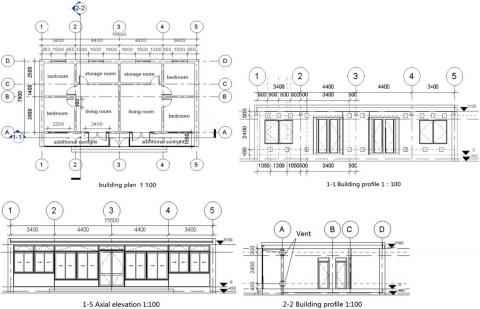Figure 1. Plan, elevation and profile of the house

Table 1. Materials and heat transfer coefficients of the envelope structure

 Components Description Heat transfer coefficient /W(m2·K)-1 External wall 30mm insulation layer + 240mm brick wall + 70mm expanded polystyrene foam board + protective layer 0.36 External window Aluminum alloy frame insulating glass (6mm + 12mm + 6mm) 2.9 Roof 200mm reinforced concrete + 90mm expanded polystyrene foam board + gypsum board ceiling 0.33 Floor 40mm gravel or pebble layer + 40mm extruded polystyrene foam board + 100mm brick concrete cushion 0.7 Sunspace Aluminum alloy frame hollow glass, aluminum alloy gusset plate for the lower part, with 30mm insulation board on the inside /

2.2 Gambit modeling

Gambit is a high-quality preprocessor for computational fluid dynamics (CFD) analysis. This software boasts a strong meshing capability, with main functions like geometric modeling and mesh generation . The dedicated meshing algorithm of the software can mesh the object into fully unstructured tetrahedral, hexahedral or mixed grids, depending on the geometry and continuity of the object. If there are additional boundary conditions, the meshed grids can be imported into ANSYS Fluent for pseudo-calculations.

In Gambit modelling, the vents of the target house are in the shape of squares. To disclose the impact of the length and number of vents on the indoor temperature and velocity fields, three different vent lengths (a=0.2m, a=0.25m, and a=0.3m), and four different vent numbers on the external wall of each bedroom (2 inlets + 1 outlet, 2 inlets + 2 outlets, 3 inlets + 2 outlets, and 3 inlets + 3 outlets) were selected for modelling. In total, 12 combinations (models) of vent length and vent number were created for simulation. The position of each vent in the vertical direction is shown in Figure 1. If there is one vent at the same height in the horizontal direction, it is arranged the middle; if there are two or three vents, their positions are arranged as shown in Figure 1.

Considering the size of the outer door of the living room, the vent number for each living room was fixed at 2 inlets + 2 outlets, with the same vent length as the bedrooms. The position of the vents is also presented in Figure 1.

Since the house is symmetrical, only the vents on the left side were considered in the simulation. For clarify, the vents in each model were numbered (Table 2).

Table 2. Serial number of the vents in each model

 No. length Amount of vents No. Length Amount of vents Mode 1 0.2m 2 inlet 1 outlet Mode 7 0.25m 3 inlet 2 outlet Mode 2 0.2m 2 inlet 2 outlet Mode 8 0.25m 3 inlet 3 outlet Mode 3 0.2m 3 inlet 2 outlet Mode 9 0.3m 2 inlet 1 outlet Mode 4 0.2m 3 inlet 3 outlet Mode 10 0.3m 2 inlet 2 outlet Mode 5 0.25m 2 inlet 1 outlet Mode 11 0.3m 3 inlet 2 outlet Mode 6 0.25m 2 inlet 2 outlet Mode 12 0.3m 3 inlet 3 outlet

The 12 models only differ in the length and number of vents. Therefore, the model of 0.3 m and 3 inlets + 2 outlets was taken as an example to illustrate the modelling process. The rural house in the model was subjected to Tet/Hybrid meshing. As shown in Figure 2, most of the house was meshed into tetrahedral grids, with hexahedral grids in a few places.

## 2.jpg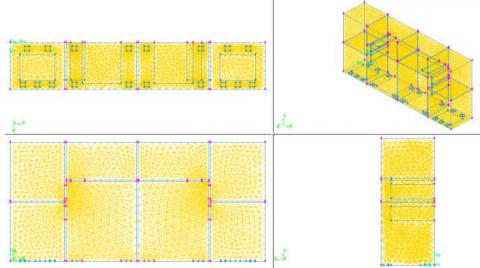Figure 2. Grid meshing of the rural house (model: 0.3m and 3 inlets + 2 outlets)

3. Fluent Simulation

3.1 Introduction to fluent

In recent years, the CFD has been widely used to solve problems in fluid mechanics and heat transfer. Fluent is one of the most popular CFD software that effectively combines classical fluid mechanics with numerical calculation methods. The cornerstone of the software is a solver based on the finite-volume method. The solution process of Fluent involves the following steps: selecting the solution model, importing the mesh file and checking the mesh, determining the calculation model and fluid mechanical properties, defining the operating environment, setting the boundary conditions, solving the parameters, initializing the flow field, setting the number of iterations, and calculating the solution.

In this paper, the rural house in each vent model is simulated on Fluent. For simplicity, the following hypotheses were put forward:

(1) Without considering the sunspace, the room temperature can be heated up 287K by the indoor heating device.

(2) The air indoor is a low-speed incompressible fluid in a turbulent flow state.

(3) According to the Boussinesq hypothesis, only the volume-dependent terms of the density in the momentum equation are considered, regardless of the viscous dissipation of the fluid.

(4) The gravity acceleration is -9.8m/s2.

(5) There is no heat transfer through radiation.

(6) The indoor furniture and personnel have no impact on the temperature field and velocity field.

(7) There is not door and window gaps on the wall of each vent.

3.2 Boundary conditions

The Fluent was used to simulate the circulation between the warm air in the sunspace and the relatively cool indoor air through the vents, i.e. the entry of the warm air into the house to raise the indoor temperature. The wind speed and temperature at each inlet was set to 0.03m/s and 300K, respectively.

The turbulence parameters at the inlet, outlet, and far-field boundaries of the computational domain were defined by “Intensity and Hydraulic Diameter” (I and DH), one of the four commonly used definition methods for turbulence parameters. The other three methods are K and Epsilon (k-ε), Intensity and Length Scale, and Intensity and Viscosity Ratio. The hydraulic diameter DH can be calculated by:

$D_{H}=\frac{F}{\chi}$

where, F is the vent area; χ is the vent perimeter.

The turbulence intensity I can be calculated by:

$I=0.16\left(R e_{D_{H}}\right)^{-1 / 8}$

where, $R e_{D_{H}}$  is the Reynolds number calculated by hydraulic diameter DH.

According to the model parameters, the outlet boundary was set to free outflow. The initial indoor temperature was set to 287K based on hypothesis (1) and the envelope structure was assumed as insulated. The heat transfer coefficient of the external walls, doors and windows adjacent to the sunspace was set to 8.7 W/(m2·K) as the third type of boundary condition. The inner wall temperature was set to 287 K, making it the first type of boundary condition. The internal door was set to free outflow .

3.3 Control equations

1. Continuity equation

$\frac{\partial u}{\partial x}+\frac{\partial v}{\partial y}+\frac{\partial w}{\partial z} 0$

2. Momentum equation

\begin{aligned} \rho\left(\frac{\partial u}{\partial t}+u \frac{\partial u}{\partial x}+v \frac{\partial u}{\partial y}+w \frac{\partial w}{\partial z}\right)=\mu\left(\frac{\partial^{2} u}{\partial x^{2}}+\frac{\partial^{2} u}{\partial y^{2}}+\frac{\partial^{2} u}{\partial z^{2}}\right)-\frac{\partial p}{\partial x} \end{aligned}

$\rho\left(\frac{\partial v}{\partial t}+u \frac{\partial v}{\partial x}+v \frac{\partial v}{\partial y}+w \frac{\partial v}{\partial z}\right)=\mu\left(\frac{\partial^{2} v}{\partial x^{2}}+\frac{\partial^{2} v}{\partial y^{2}}+\frac{\partial^{2} v}{\partial z^{2}}\right)-\frac{\partial p}{\partial y}$

\begin{aligned} \rho\left(\frac{\partial w}{\partial t}+u \frac{\partial w}{\partial x}+v \frac{\partial w}{\partial y}+w \frac{\partial w}{\partial z}\right)\mu\left(\frac{\partial^{2} w}{\partial x^{2}}+\frac{\partial^{2} w}{\partial y^{2}}+\frac{\partial^{2} w}{\partial z^{2}}\right)-\frac{\partial p}{\partial z} \end{aligned}

3. Energy equation

$\frac{\partial T}{\partial t}+u \frac{\partial T}{\partial x}+v \frac{\partial T}{\partial y}+w \frac{\partial T}{\partial z}=\alpha\left(\frac{\partial^{2} T}{\partial x^{2}}+\frac{\partial^{2} T}{\partial y^{2}}+\frac{\partial^{2} T}{\partial z^{2}}\right)$

4. k, ε and viscosity coefficient equations

$\rho \frac{\partial k}{\partial t}+\rho u_{j} \frac{\partial k}{\partial x_{j}}=\left[\left(\eta+\frac{\eta_{t}}{\sigma_{\varepsilon}}\right) \frac{\partial \varepsilon}{\partial x_{k}}\right]+\eta_{t} \frac{\partial u}{\partial x_{i}}\left(\frac{\partial u_{i}}{\partial x_{j}}+\frac{\partial u_{j}}{\partial x_{i}}\right)-\rho \varepsilon$

\begin{aligned} \rho \frac{\partial k}{\partial t}+\rho u_{k} \frac{\partial}{\partial x_{k}} &=\left[\left(\eta+\frac{\eta_{t}}{\sigma_{\varepsilon}}\right) \frac{\partial \varepsilon}{\partial x_{k}}\right]\frac{c_{1} \varepsilon}{k} \eta_{t} \frac{\partial u_{i}}{\partial x_{i}}\left(\frac{\partial u_{i}}{\partial x_{j}}+\frac{\partial u_{j}}{\partial x_{i}}\right)-c_{2} \rho \frac{\varepsilon^{2}}{k} \end{aligned}

\begin{aligned} \eta_{t}=c_{\mu} \rho k^{\frac{1}{2}} l=\left(c_{\mu}^{\prime} c_{D}\right) \rho k^{2} & \frac{1}{c_{D} k^{\frac{3}{2}} / l}=c_{\mu} \rho k^{2} / \varepsilon \\ c_{\mu}=& c_{\mu}^{\prime} c_{D} \end{aligned}

where, x, y and z are the three directions in the Cartesian coordinate system (m); u, v and w are the velocities in the three directions, respectively (m/s); t is time (s); ρ is density (kg/m2); μ is dynamic viscosity (N·s/m2); P is pressure (Pa); T is temperature (°C); α is thermal diffusivity (m2/s).

Three coefficients (c1, c2 and cμ) and three constants (σk, σεand σT) were introduced into the above equations. The values of these six empirical coefficients are shown in Table 3.

Table 3. Coefficients in the k-ε model

 cμ c1 c2 σk σε σT 0.09 1.44 1.92 1.0 1.3 0.9~1.0
4. Calculation Results

Since the house is symmetrical, our simulation only covers the bedroom and the living room on the left side. According to the relevant provisions in the Building Energy Conservation Technology, six measuring points were set up on the intersection of plane y=2m and plane z=1.5m, and another six on the intersection plane y=2m and plane z=2m, including (0.8, 2, 1.5), (1.6, 2, 1.5), (2.4, 2, 1.5), (4.6, 2, 1.5). , (5.7, 2, 1.5), (6.8, 2, 1.5), (0.8, 2, 2), (1.6, 2, 2), (2.4, 2, 2), (4.6, 2, 2), ( 5.7, 2, 2) and (6.8, 2, 2). During the monitoring, it is found that the temperature at each measuring point initially increased at a fast speed and tended to be stable in 2h. The temperature trend of each model in 2h is provided in Figure 3 below.

## 4.jpg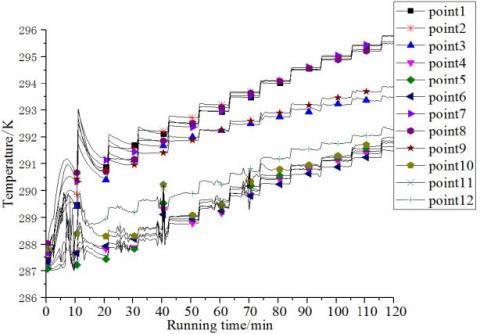Figure 3. Temperature trend of each model in 2h

As shown in Figure 3, the temperature of each model at the heights of 1.5 m and 2 m in the house tended to be the same, whether in the bedroom or the living room. Hence, it is only necessary to analyze the data at z=1.5 m. Then, the models were sorted in descending order of temperature (Table 4).

Table 4. Temperature ranking of the models

 Ranking Bedroom Living room Ranking Bedroom Living room 1 Model 11 Model 11 7 Model 3 Model 6 2 Model 12 Model 2 8 Model 6 Model 7 3 Model 8 Model 12 9 Model 5 Model 4 4 Model 7 Model 10 10 Model 4 Model 5 5 Model 9 Model 9 11 Model 2 Model 1 6 Model 10 Model 8 12 Model 1 Model 3
5. Comparison of Temperature Field and Velocity Field

The simulation results show that Model 11 (0.3m and 3 inlets + 2 outlets) and model 1 (0.2m and 2 inlets + 1 outlet) had the best and worst performance, respectively. Therefore, the two models were further compared in temperature field and velocity field.

5.1 Temperature field analysis

The hourly temperature change at each measuring point was monitored in 2h. Based on the collected data, the temperature trend of each measuring point was potted (Figures 4 and 5).

## 4.jpgFigure 4. Temperature curve of measuring points of model 11

## 5.jpg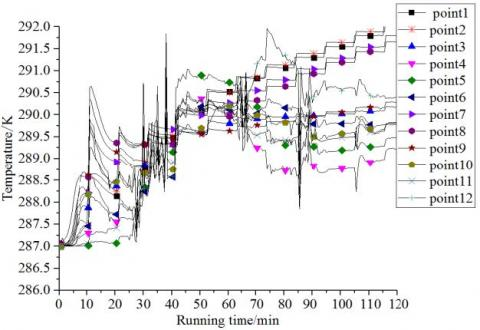Figure 5. Temperature curve of measuring points of model 1

## 6.jpg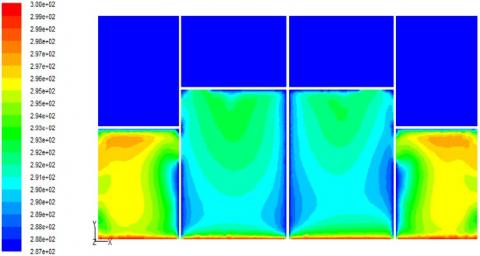Figure 6. Temperature distribution in section Z=1.5m of model 11

## 7.jpg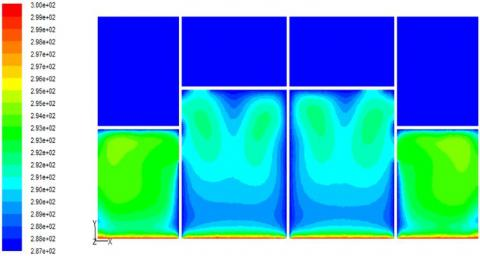Figure 7. Temperature distribution in section Z=1.5m of model 1

## 8.jpg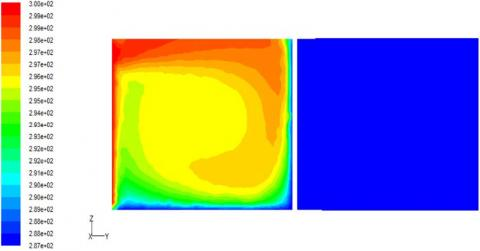Figure 8. Temperature distribution in section X=1.6m of model 11

## 9.jpg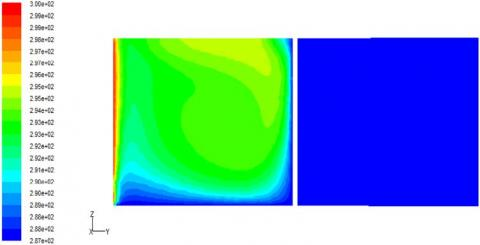Figure 9. Temperature distribution in section X=1.6m of model 1

As shown in Figures 4 and 5, the temperature of each measuring point rose at a decreasing rate with the elapse of time. The hourly temperature of model 11 in bedroom was 4~5K higher than that of model 1. Next, the cloud map of temperature distribution of the two models were prepared based on the data of sections z=1.5 m and x=1.6 m.

It can be seen that the rooms adjacent to the sunspace were affected by the sunspace temperature more than the other rooms (See Figures 6-9). Despite having the same number of vents, the living room was cooler than the bedroom, due to its relatively large volume. According to the maps about the section x=1.6m, the temperature in the upper part of the room was significantly higher than that in the lower part. This is because the warm air enters through the upper inlet, while the cold air flows out through the lower outlet. Overall, the temperature field of model 11 was much more uniform than that of model 1, and the overall temperature of the former was also higher than that of the latter.

5.2 Velocity field analysis

The temperature and comfort of a room are affected by the air distribution. Here, the velocity distribution is analyzed based on the vertical sections x=1.6m and x=6.8m, which are the middle of the bedroom and the living room, respectively (Figures 10-13).

It can be seen that stable vortices appeared in the vertical sections of both models, but the velocity distribution of model 1 was much weaker than that of model 11. Thus, model 1 had a much poorer air circulation than model 11. Then, the velocity distribution at the horizontal section z=1.5m in each model was plotted (Figures 14 and 15).

It can be seen that a stable eddy current was formed in model 11. By contrast, the temperature of model 1 was relatively low, because the air circulation and heat transfer are suppressed by the low air velocity.

## 10.jpg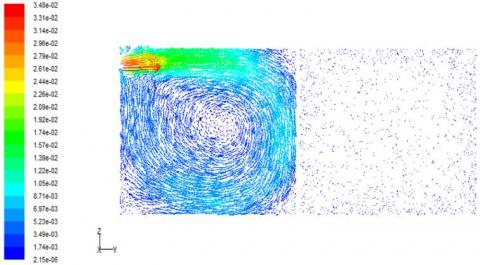Figure 10. Velocity distribution of vertical section X=1.6m in model 11

## 11.jpg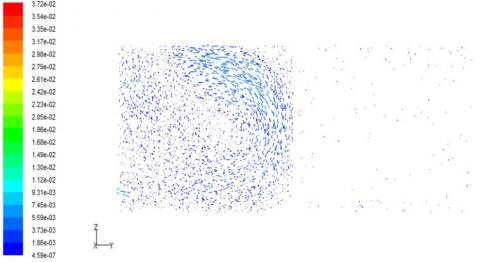Figure 11. Velocity distribution of vertical section X=1.6m in model 1

## 12.jpg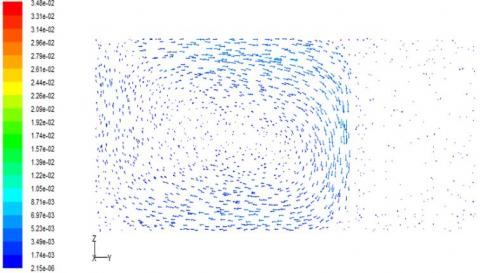Figure 12. Velocity distribution of vertical section X=6.8m in model 11

## 13.jpg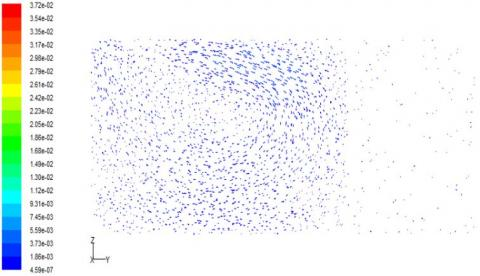Figure 13. Velocity distribution of vertical section X=6.8m in model 1

## 14.jpg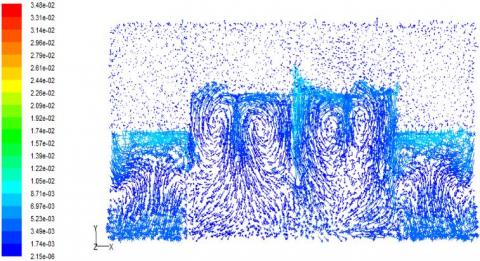Figure 14. Velocity distribution in horizontal section Z=1.5m of model 11

## 15.jpg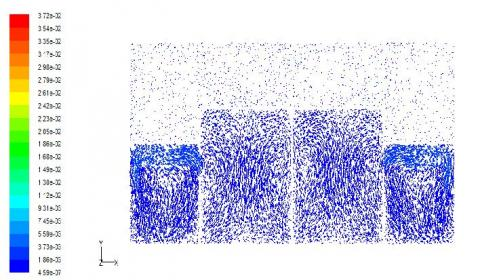Figure 15. Velocity distribution in horizontal section Z=1.5m of model 1

5.3 Correlation analysis of temperature field and velocity field

The analysis in Subsections 5.1 and 5.2 demonstrates that model 11 had better temperature field and velocity field than model 1. As mentioned before, the heat exchange between the sunspace and the indoor mainly exists in the form of convection: the hot air moves from the sunspace to the room via the upper vent, while the cold air moves from the indoor to the sunspace from the lower vent. With the better air circulation and heat transfer efficiency, model 11 enjoys a higher temperature than model 1.

6. Environmental Benefit Analysis

The pollution of winter heating is a longstanding environmental problem in rural China. The target rural house was subjected to load calculation on DeST, an integrated building simulation toolkit, before energy-saving renovation. The results show that the heating load index was 84.8W/m2, and the cumulative load during the heating season was 37,939.75kW·h. Since the efficiency of heating furnace is mostly 45~55% and the heating season lasts 122 days, the coal consumption of the target house is about 8.5t, equivalent to 9.3t of standard coal.

After the energy-saving renovation by our vent model, the heating load index was reduced to 37.2W/m2 and the cumulative load during the heating season was lowered to 13,595.49kW·h, saving 64% of energy (5.95t of standard coal). One ton of standard coal could emit 2.66t-2.72t of carbon dioxide. Hence, the renovation helps to suppress the carbon dioxide emissions by 15.8t-16.2t per year. Therefore, our vent design could greatly improve the ecological environment in rural areas, and reduce the burden of ecological environment.

7. Conclusions

This paper analyzes the temperature field and velocity field of 12 vent models, and further compares the temperature and velocity distributions of the best and worst performing models. Considering the relative error of Fluent simulation, the author drew the following conclusions:

(1) Under the same number of vents, the longer the vent, the higher the indoor temperature. Under the same length of vent, the highest indoor temperature belongs to the models of 3 inlets and 2 outlets. Out of all the models, model 11 (0.3m and 3 inlets + 2 outlets) achieved the highest indoor temperature.

(2) The room volume and depth affect how the vent length and vent number influences the indoor temperature. The length and number of vents can affect the indoor air circulation. The optimal inlet-outlet ratio for heat transfer is 3:2. Our results show that, the longer the vent length, the faster the indoor air velocity, and the better the heat transfer.

(3) The proposed vent design can greatly reduce energy consumption and carbon emissions, thus improving the ecological environment. In the rural areas of Hebei province, the sunspace design should select high-performance low-cost glasses to reduce the construction cost, and optimize the number and length of the vents in the sunspace based on our simulation data.

In this research, the vents are assumed to be square in shape. The other shapes were not taken into account. Therefore, the future research will study the vents in multiple other shapes, compare the simulation results with the data in this paper, and further analyze the relationship between the heat supply of new heating mode and the heat load of the renovated rural house.

Acknowledgements

This research was supported by Shijiazhuang Science and Technology Research and Development Project (Soft Science) - Research on Application and Promotion of Existing Building Energy Saving and Solar Heating Mode in Rural Areas--Taking Shijiazhuang as an Example (185230055A); and the Key R&D Program of Hebei.

References

 He, B., Yang, L., Ye, M. (2014). Building energy efficiency in China rural areas: Situation, drawbacks, challenges, corresponding measures and policies. Sustainable Cities and Society, 11: 7-15. https://doi.org/10.1016/j.scs.2013.11.005

 Xiong, K., Yang, Z., Ran, J. (2017). Research on passive energy-saving renovation of the roof of traditional residences. Energy Procedia, 141: 240-244. https://doi.org/10.1016/j.egypro.2017.11.099

 Xiong, K., Yang, Z. (2017). Energy-saving renovation of Bayu traditional residence: Taking Anju Town of Chongqing as the example. Procedia Engineering, 180: 687-696. https://doi.org/10.1016/j.proeng.2017.04.228

 Buvik, K., Andersen, G., Tangen, S. (2015). Energy upgrading of a historical school building in cold climate. Energy Procedia, 78: 3342-3347. https://doi.org/10.1016/j.egypro.2015.11.748

 Chwieduk, D. (2016). Some aspects of energy efficient building envelope in high latitude countries. Solar Energy, 133: 194-206. https://doi.org/10.1016/j.solener.2016.03.068

 Liu, Z.J., Liu, Y.W., He, B.J., Xu, W., Jin, G.Y., Zhang, X.T. (2019). Application and suitability analysis of the key technologies in nearly zero energy buildings in China. Renewable and Sustainable Energy Reviews, 101: 329-345. https://doi.org/10.1016/j.rser.2018.11.023

 Dermentzis, G., Ochs, F., Siegele, D., Feist, W. (2018). Renovation with an innovative compact heating and ventilation system integrated into the façade-An in-situ monitoring case study. Energy and Buildings, 165: 451-463. https://doi.org/10.1016/j.enbuild.2017.12.054

 Chen, X., Yang, H.X. (2018). Integrated energy performance optimization of a passively designed high-rise residential building in different climatic zones of China. Applied Energy, 215: 145-158. https://doi.org/10.1016/j.apenergy.2018.01.099

 Ekström, T., Bernardo, R., Blomsterberg, Å. (2018). Cost-effective passive house renovation packages for Swedish single-family houses from the 1960s and 1970s. Energy and Buildings, 161: 89-102. https://doi.org/10.1016/j.enbuild.2017.12.018.

 Mihai, M., Tanasiev, V., Dinca, C., Badea, A., Vidu, R. (2017). Passive house analysis in terms of energy performance. Energy and Buildings, 144: 74-86. https://doi.org/10.1016/j.enbuild.2017.03.025.

 Li, G.Q., Xuan, Q.D., Lu, Y.S., Pei, G., Su, Y., Ji, J. (2018). Numerical and lab experiment study of a novel concentrating PV with uniform flux distribution. Solar Energy Materials and Solar Cells, 179: 1-9. https://doi.org/10.1016/j.solmat.2018.02.012

 Schnieders, J., Feist, W., Rongen, L. (2015). Passive houses for different climate zones. Energy and Buildings, 105: 71-87. https://doi.org/10.1016/j.enbuild.2015.07.032

 Zirnhelt, H.E., Richman, R.C. (2015). The potential energy savings from residential passive solar design in Canada. Energy and Buildings, 103: 224-237. https://doi.org/10.1016/j.enbuild.2015.06.051

 de Rubeis, T., Nardi, I., Ambrosini, D., Paoletti, D. (2018). Is a self-sufficient building energy efficient? Lesson learned from a case study in Mediterranean climate. Applied Energy, 218: 131-145. https://doi.org/10.1016/j.apenergy.2018.02.166

 Ge, T.S., Wang, R.Z., Xu, Z.Y., Pan, Q.W., Du, S., Chen, X.M., Ma, T., Wu, X.N., Sun, X.L., Chen, J.F. (2018). Solar heating and cooling: present and future development. Renewable Energy, 126: 1126-1140. https://doi.org/10.1016/j.renene.2017.06.081

 Mussard, M. (2017). Solar energy under cold climatic conditions: a review. Renewable and Sustainable Energy Reviews, 74: 733-745. https://doi.org/10.1016/j.rser.2017.03.009.

 Rabani, M., Kalantar, V., Dehghan, A., Faghih, A. (2015). Experimental study of the heating performance of a Trombe wall with a new design. Solar Energy, 118: 359-374. https://doi.org/10.1016/j.solener.2015.06.002

 Du, X.H., Jia, B. (2019). Discussion on applying trombe wall technology for wall conservation and energy saving in modern historic buildings. International Journal of Architectural Heritage, 13(4): 537-548. http://doi.org/10.1080/15583058.2018.1440029

 Nikolić, D., Skerlić, J., Cvetković, D., Radulović, J., Jovanović, S. (2018). Basic principles of passive solar heating. In 3rd International conference on Quality of Life, Kopaonik, Serbia, 28-30.

 Ma, Q., Fukuda, H., Lee, M., Kobatake, T., Kuma, Y., Ozaki, A., Wei, X. (2018). Study on heat utilization in an attached sunspace in a house with a central heating, ventilation, and air conditioning system. Energies, 11(5): 1192-1203. https://doi.org/10.3390/en11051192

 Zhao, F.Y., Liu, D., Wang, H.Q., Kou, G.X., Tang, G.F. (2010). Free heat and mass transfer in a porous enclosure with side vents. Drying Technology, 29(1): 91-104. http://doi.org/10.1080/07373937.2010.482701

 Evans, M., Yu, S., Song, B., Deng, Q.Q., Liu, J., Delgado, A. (2014). Building energy efficiency in rural China. Energy Policy, 64: 243-251. http://doi.org/10.1016/j.enpol.2013.06.040

 Kendouci, M., Kharroubi, B., Khelfaoui, R., Bendida, A., Dennai, B., Maazouzi, A. (2013). Simulation of water filtration in porous zone based on darcy's law. Energy Procedia, 36: 163-168. https://doi.org/10.1016/j.egypro.2013.07.019

 Mirsadeghi, M., Cóstola, D., Blocken, B., Hensen, J. L. (2013). Review of external convective heat transfer coefficient models in building energy simulation programs: Implementation and uncertainty. Applied Thermal Engineering, 56(1-2): 134-151. http://doi.org/10.1016/j.applthermaleng.2013.03.003